• int NInorderTraverse(BiTree &Tint*visit(char e) { InitStack(S; Push(S,T;//根指针入栈 while!StackEmpty(S)//在树不空情况下 { while(GetTop(S,p&p) Push(S,p->...//空指针退 if!StackEmpty(S) { Pop(S,p;
• 前言 Day04介绍了Reverse Polish Notation，主要是为了方便用这个结构计算数学表达式。...流程图 主要代码 - (int)evaluateRPNNSString *)inputStr { DSStack *newStack = [...
前言

Day04介绍了Reverse Polish Notation，主要是为了方便用栈这个结构计算数学表达式。复习下：

输入前：（3 + 2）*（4 + 6）转化后：3 2 + 4 6 + *结果：50

流程图主要代码

- (int)evaluateRPNNSString *)inputStr

{

   DSStack *newStack = [[DSStack alloc] initWithSize:10];

   for (int i =0 ; i<inputStr.length; i++)

   {

       unichar tempChar = [inputStr characterAtIndex:i];

       if ([[NSString stringWithCharacters:&tempChar length:1] isEqualToString:@"*"])

       {

           NSNumber *a = [newStack popLastObject];

           NSNumber *b = [newStack popLastObject];

           [newStack push:[NSNumber numberWithInt:([a intValue]*[b intValue])]];

       }

       else if ([[NSString stringWithCharacters:&tempChar length:1] isEqualToString:@"/"])

       {

           NSNumber *a = [newStack popLastObject];

           NSNumber *b = [newStack popLastObject];

           [newStack push:[NSNumber numberWithInt:([a intValue]/[b intValue])]];

       }

       else if ([[NSString stringWithCharacters:&tempChar length:1] isEqualToString:@"+"])

       {

           NSNumber *a = [newStack popLastObject];

           NSNumber *b = [newStack popLastObject];

           [newStack push:[NSNumber numberWithInt:([a intValue]+[b intValue])]];

       }

       else if ([[NSString stringWithCharacters:&tempChar length:1] isEqualToString:@"-"])

       {

           NSNumber *a = [newStack popLastObject];

           NSNumber *b = [newStack popLastObject];

           [newStack push:[NSNumber numberWithInt:([a intValue]-[b intValue])]];

       }

       else

       {

           [newStack push:[NSNumber numberWithInt:[[NSString stringWithCharacters:&tempChar length:1] intValue]]];

       }

   }

   return [[newStack popLastObject] intValue];

}

大致思路描述

把中缀表达式转化为Reverse Polish Notation。

按照顺序进栈。

当进栈的元素是 （ + – * / ）四个运算符，则弹出栈顶两个元素并计算，并且把结果入栈。

最终栈只剩下一个元素，这个元素就是最后的值。

100天iOS数据结构与算法实战 Day01

100天iOS数据结构与算法实战 Day02 – 栈

100天iOS数据结构与算法实战 Day03 – 栈的算法实战 Valid Parentheses

100天iOS数据结构与算法实战 Day04 – 栈的算法实战 逆波兰表示法

References

交流群昵称：ios-Swift/Object C开发上架
交流群号： 869685378   找ios马甲包开发者合作，有兴趣请添加Q 51259559

展开全文• 算法流程图 队列 代码实现 #include<iostream> #include<stack> #include<queue> using namespace std; class Node { public: Node(int a=0,int b=0,int c=0):x(a),y(b),di(c){} int x; ...
算法流程图
栈队列代码实现
#include<iostream>
#include<stack>
#include<queue>
using namespace std;
class Node
{
public:
Node(int a=0,int b=0,int c=0):x(a),y(b),di(c){}
int x;
int y;
int di;
};
int map = { {1,1,1,1,1,1,1,1,1,1,1,1,1,1,1},   //示例迷宫，起点（1,1），终点（13,13）
{1,0,1,1,1,1,1,1,1,1,1,1,1,1,1},
{1,0,0,1,1,1,1,1,0,0,0,0,1,1,1},
{1,1,0,1,1,1,1,1,0,1,1,0,1,1,1},
{1,1,0,1,1,1,1,1,1,1,1,0,1,1,1},
{1,1,0,0,0,0,0,0,0,0,0,0,1,1,1},
{1,1,1,1,1,0,1,1,1,1,1,0,1,1,1},
{1,1,1,1,1,0,0,1,1,1,0,0,1,1,1},
{1,1,1,1,1,1,0,0,1,1,0,1,1,1,1},
{1,1,1,1,1,1,1,0,1,1,0,1,1,1,1},
{1,1,1,1,0,0,0,0,1,1,0,1,1,1,1},
{1,1,1,1,1,1,1,0,1,1,0,1,1,1,1},
{1,1,1,1,0,1,1,0,1,1,1,1,1,1,1},
{1,1,1,1,0,0,0,0,0,0,0,0,0,0,1},
{1,1,1,1,1,1,1,1,1,1,1,1,1,1,1}};
int passed = { 0 };      //判断矩阵
bool PassC(int x, int y)
{
if (!map[x][y] && !passed[x][y])
return true;
else
return false;
}
Node NextNode(Node temp)
{
switch (temp.di)
{
case 1:
temp.y++;
break;
case 2:
temp.x++;
break;
case 3:
temp.y--;
break;
case 4:
temp.x--;
break;
default:
break;
}
temp.di = 1;
return temp;
}
bool MazePathStack(stack<Node>& temp)   //栈
{
Node present(1, 1, 1);//
while (true)
{
if (PassC(present.x, present.y))
{
temp.push(present);
passed[present.x][present.y] = 1;
if (present.x == 13 && present.y == 13)
return true;
present=NextNode(present);
}
else
{
while (!temp.empty())
{
Node *t = &temp.top();
if (t->di != 4)
{
t->di++;
present = NextNode(*t);
break;
}
else
temp.pop();
}
if (temp.empty())
return false;
}
}
}
bool MazePathQueue(queue<Node>&temp)    //队列
{

Node present(1, 1, 1);//
while (true)
{
if (PassC(present.x, present.y))
{
temp.push(present);
passed[present.x][present.y] = 1;
if (present.x == 13 && present.y == 13)
return true;
present = NextNode(present);
}
else
{
while (!temp.empty())
{
Node *t = &temp.back();
if (t->di != 4)
{
t->di++;
present = NextNode(*t);
break;
}
else
{
queue<Node> newq;
while (!(temp.front().x == t->x && temp.front().y == t->y))
{
newq.push(temp.front());
temp.pop();
}
temp.pop();
temp = newq;
}
}
if (temp.empty())
return false;
}
}
}
int main()
{
stack<Node>path;
queue<Node>Path;
Node point;
int choice;
cout << "栈实现输入1，队列实现输入2";
cin >> choice;
if (choice == 1)
{
if (MazePathStack(path))   //栈
while (!path.empty())
{
point = path.top();
cout << ++point.x << ":" << ++point.y << ",";
path.pop();
}
else
cout << "路径不存在！";
}
else
{
if (MazePathQueue(Path))  //队列
{
while (!Path.empty())
{
point = Path.front();
cout << ++point.x << ":" << ++point.y << ",";
Path.pop();
}
}
else
cout << "路径不存在！";
}
return 0;
}



展开全文c++ 队列
• 栈的思想就是先进后出，就跟堆盘子一样，先堆的在下面，先拿后放的。 流程如下所示： 代码如下： public class aa { static class queues{ //模拟结构体方便扩展 static int datas = 100; //队列的主体用来...
栈：
栈的思想就是先进后出，就跟堆盘子一样，先堆的在下面，先拿后放的。
流程如下图所示：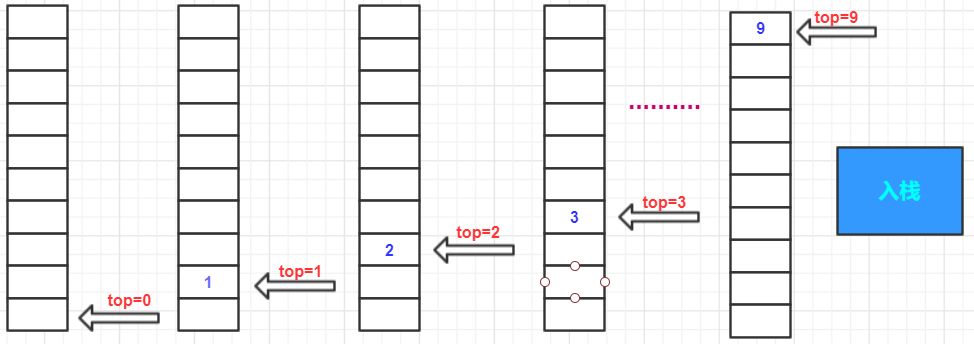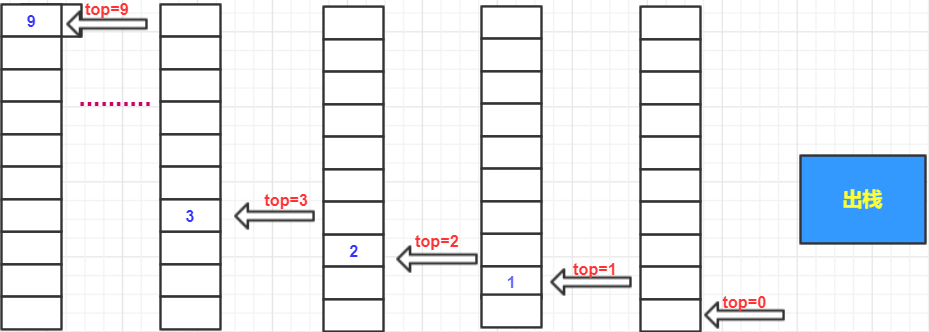代码如下：

public class aa {

static  class  queues{   //模拟结构体方便扩展
static int datas = 100; //队列的主体用来存储内容
static int top;  //栈顶
}

static void queue(int [] list){  //实现方法
int []a = new int[queues.datas]; //初始化存储体大小
queues.top = 0;  //设置栈顶只能从栈顶出入数据
//入栈
for(int i = 0;i < list.length;i++){
queues.top++;   //栈底0不存数据  所以要先+
a[queues.top] = list[i];  //从栈顶人栈
}
System.out.print("出栈顺序：");
// 出栈
while (queues.top >0){// 当对栈不为空的时候执行循环
System.out.print(a[queues.top]);//从栈顶出栈
queues.top--;
}
}
public static void main(String[] args) {
int[] s = {1,2,3,4,5,6,7,8,9};
System.out.print("入栈顺序：");
for(int i :s){
System.out.print(i);
}
System.out.println();
aa.queue(s);
}
}


结果如下图所示：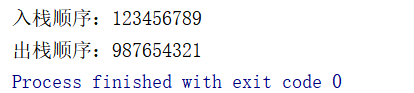展开全文• 文章目录题目数据定义程序各函数主要思想流程图输出分析源码暴力和简单贪心 马踏棋盘是栈的一个十分经典的应用，最基本的完成思路其实就是深度优先搜索(dfs)，是一种十分暴力的处理方式，费时费力还不一定可以得到一...
文章目录题目数据定义程序各函数主要思想流程图输出分析源码暴力和简单贪心
马踏棋盘是栈的一个十分经典的应用，最基本的完成思路其实就是深度优先搜索(dfs)，是一种十分暴力的处理方式，费时费力还不一定可以得到一个好的结果。使用贪心算法，将每一步，每一步的下一步都进行贪心，便会节省大量的时间，而且成功率十分客观，现就马踏棋盘的一种贪心算法做以下总结
题目
设计一个国际象棋的马踏遍棋盘的演示程序。将马随机放在国际象棋 8x8 棋盘 Board的某个方格中，马按走棋规则进行移动。要求每个方格只进入一次，走遍棋盘上全部 64 个方格。编制递归或非递归程序，求出马的行走路线，并按求出的行走路线输出。
（1）将行走路线以坐标的形式输出。
（2）在方阵中输出 1~64 个行走足迹；
数据定义
#include<stdio.h>
#include<stdlib.h>
#include<time.h>
#define ROW 8
#define COL 8
#define MAX_STEPS ROW*COL

//栈结构体
typedef struct stack{
int x_adr;      //纵坐标
int y_adr;      //横坐标
int direction;  //方向
}HORSE_CHESS_STACK;

//存储路径棋盘
int chess[ROW+1][COL+1];

//下一步方向
//一号
int dir = {{2,-1},{-2,-1},{-2,1},{2,1},
{1,-2},{-1,-2},{-1,2},{1,2}};
//二号
/* int dir = {{1,2},{-1,-2},{-2,1},{2,1},
*                  {2,-1},{1,-2},{-1,2},{-2,-1}}; */

//栈顶
int top;
HORSE_CHESS_STACK Adr_Stack[MAX_STEPS];

//出栈次数
int out_stack;

值得注意的是，结构体中，x_adr 其实是真实的纵坐标，y_adr 其实是真实的横坐标（大家画个数组表示的棋盘，其实就懂了）。这个首先要明确，不然在后面的定义和赋值等操作时，会有很多不便。
程序各函数
//初始化数据
void init();
//debug 打印栈的情况
void print_stack();
//入栈
void push_stack(int x_real,int y_real);
//出栈
void pop_stack();
//标记位置
void mark_chess(int x,int y);
//打印路径
void print_chess_board();
//打印每一步的位置
int t = 1;
void print_steps();
//马踏棋盘（贪心）算法
void run_horse_tanxin();

主要思想
我们算法最核心的部分，是在下一步到底应该如何选择这件事上，主要的贪心思路就是：
我们先判断下一步都有哪些位置可以走，然后我们再判断其可走位置的再下一步，有几个位置可以走，并统计这几个末端位置的在下一步有几个位置可以走。
也就是 分别计算当前位置下下一步的权，之后我们将下下一步的权都加起来，算成下一步的权，并存储到 next 数组里面。
之后，我们建立 real_next 数组，通过查找遍历，依次将 next 中最小元素的下标赋值给 real_next 数组，这样，我们就得到了一个下一步走的方向顺序的数组 real_next。
这时，我们就可以开始按照 real_next 的顺序来走下一步
以下是核心代码(注释已加)：
		//现在位置
x_now = Adr_Stack[top].x_adr;
y_now = Adr_Stack[top].y_adr;

//对方向进行排序
int next[ROW] = {};
for(int i = 0;i < ROW;i++){
//下一步坐标
int x_next = x_now + dir[i];
int y_next = y_now + dir[i];

if((x_next > 0 && x_next <= COL) && (y_next > 0 && y_next <= ROW) && chess[y_next][x_next] == 0 ){
//对下一步的下一步判断是否可以走
for(int j = 0;j < ROW;j++){
int x_next_next = x_next + dir[j];
int y_next_next = y_next + dir[j];

//可以走,next 对应下标+1
if((x_next_next > 0 && x_next_next <= COL) && (y_next_next > 0 && y_next_next <= ROW) && chess[y_next_next][x_next_next] == 0){
next[i]++;
}
}
}
}

//依次返回 next 中最小元素的下标，返回后将元素赋值为最大
int real_next = {0};
int k = 0;
int t = ROW + 1;
for(int i = 0;i < ROW;i++){
t = ROW + 1;
for(int j = 0;j < 8;j++){
if(next[j] < t){
real_next[i] = j;
t = next[j];
k = j;
}
}
next[k] = ROW + 1;
}

//走下一步
int dir_now = 0;
for(dir_now = Adr_Stack[top].direction + 1;dir_now < ROW;dir_now++){
int x_real = x_now + dir[real_next[dir_now]];
int y_real = y_now + dir[real_next[dir_now]];

Adr_Stack[top].direction += 1;

if((x_real <= COL && x_real > 0) && (y_real <= ROW && y_real > 0) && chess[y_real][x_real] == 0){
//入栈
push_stack(x_real,y_real);
//标记棋盘
mark_chess(x_real,y_real);
break;
}
}

流程图输出分析
下面环境是Linux Ubuntu 18.04
经过一系列实验输入，最终64个位置，无论是从哪个开始，都可以走完全部棋盘，且大部分情况下，没有出栈回溯的情况
以下是一号方向数组在起始位置是（1,1）所得出的答案：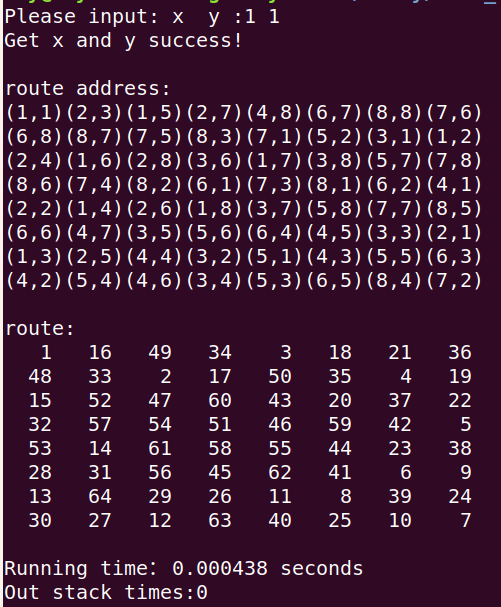在一号方向数组中，只有在 8,6这个位置，有回溯发生：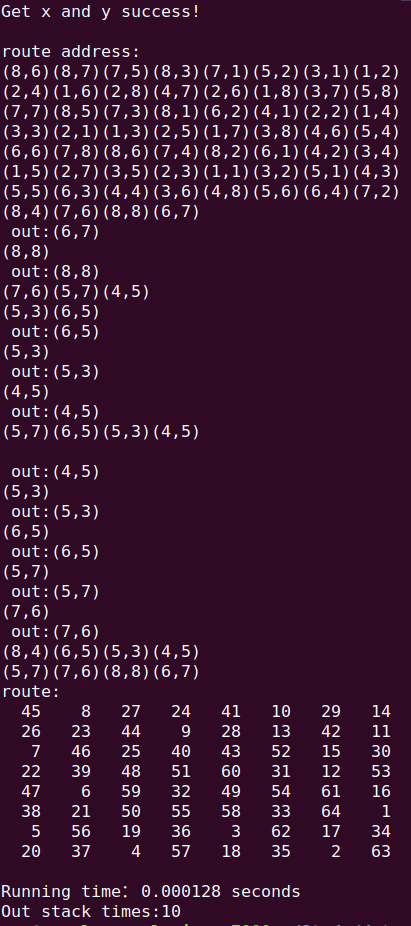我们还应该注意的是，当方向数组的顺序定义得不同时，得到的走的方案也很大可能是不同的
下面，是二号方向数组数组的同样 （1 1）位置所得出的路径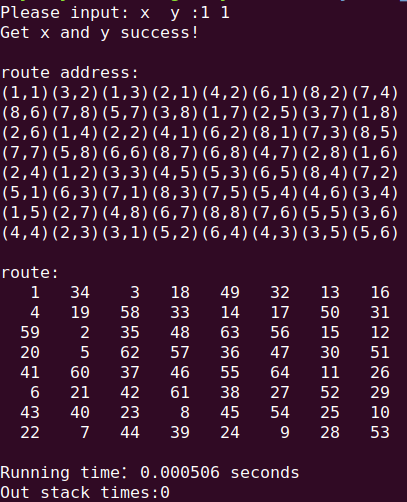同时我们通过观察其用时发现，贪心的效率十分可观
源码
注释已加
#include<stdio.h>
#include<stdlib.h>
#include<time.h>
#define ROW 8
#define COL 8
#define MAX_STEPS ROW*COL

//栈结构体
typedef struct stack{
int x_adr;      //纵坐标
int y_adr;      //横坐标
int direction;  //方向
}HORSE_CHESS_STACK;

//存储路径棋盘
int chess[ROW+1][COL+1];

//下一步方向
//一号
int dir = {{2,-1},{-2,-1},{-2,1},{2,1},
{1,-2},{-1,-2},{-1,2},{1,2}};

//二号
/* int dir = {{1,2},{-1,-2},{-2,1},{2,1},
{2,-1},{1,-2},{-1,2},{-2,-1}}; */

//栈顶
int top;
HORSE_CHESS_STACK Adr_Stack[MAX_STEPS];

//出栈次数
int out_stack;

//初始化数据
void init(){
int n = MAX_STEPS;
while(n--){
Adr_Stack[n].x_adr = 0;
Adr_Stack[n].y_adr = 0;
Adr_Stack[n].direction = -1;
}
Adr_Stack.x_adr = 0;
Adr_Stack.y_adr = 0;
Adr_Stack.direction = -1;

for(int i = 1;i <= ROW;i++){
for(int j = 1;j <= COL;j++){
chess[ROW][COL] = 0;
}
}

top = -1;
out_stack = 0;
}

//debug 打印栈的情况
void print_stack(){
printf("栈的情况:\n");
for(int i = 0;i < MAX_STEPS;i++){
printf("x:%d  y:%d direction = %d\n",Adr_Stack[i].y_adr,Adr_Stack[i].x_adr,Adr_Stack[i].direction);
}
printf("\n\n");
}

//入栈
void push_stack(int x_real,int y_real){
top++;
Adr_Stack[top].x_adr = x_real;
Adr_Stack[top].y_adr = y_real;
Adr_Stack[top].direction = -1;  //初始化走的方向
}

//出栈
void pop_stack(){
Adr_Stack[top].x_adr = 0;
Adr_Stack[top].y_adr = 0;
Adr_Stack[top].direction = -1;  //初始化走的方向
top--;
}

//标记位置
void mark_chess(int x,int y){
chess[y][x] = top + 1;
}

//打印路径
void print_chess_board(){
printf("\nroute:\n");
for(int i = 1;i <= ROW;i++){
for(int j = 1;j <= ROW;j++){
printf("%4d ",chess[i][j]);
}
printf("\n");
}
printf("\n");
}

//打印每一步的位置
int t = 1;
void print_steps(){
printf("(%d,%d)",Adr_Stack[top].y_adr,Adr_Stack[top].x_adr);
t++;
if(t == ROW){
printf("\n");
t = 0;
}
}

//马踏棋盘（贪心）算法
void run_horse_tanxin(){
int x_now,y_now;
while(1){

//已经走完全图
if(top >= MAX_STEPS - 1){
//打印棋盘
print_chess_board();
break;
}

//现在位置
x_now = Adr_Stack[top].x_adr;
y_now = Adr_Stack[top].y_adr;

//对方向进行排序
int next[ROW] = {};
for(int i = 0;i < ROW;i++){
//下一步坐标
int x_next = x_now + dir[i];
int y_next = y_now + dir[i];

if((x_next > 0 && x_next <= COL) && (y_next > 0 && y_next <= ROW) && chess[y_next][x_next] == 0 ){
//对下一步的下一步判断是否可以走
for(int j = 0;j < ROW;j++){
int x_next_next = x_next + dir[j];
int y_next_next = y_next + dir[j];

//可以走,next 对应下标+1
if((x_next_next > 0 && x_next_next <= COL) && (y_next_next > 0 && y_next_next <= ROW) && chess[y_next_next][x_next_next] == 0){
next[i]++;
}
}
}
}

//依次返回 next 中最小元素的下标，返回后将元素赋值为最大
int real_next = {0};
int k = 0;
int t = ROW + 1;
for(int i = 0;i < ROW;i++){
t = ROW + 1;
for(int j = 0;j < 8;j++){
if(next[j] < t){
real_next[i] = j;
t = next[j];
k = j;
}
}
next[k] = ROW + 1;
}

//走下一步
int dir_now = 0;
for(dir_now = Adr_Stack[top].direction + 1;dir_now < ROW;dir_now++){
int x_real = x_now + dir[real_next[dir_now]];
int y_real = y_now + dir[real_next[dir_now]];

Adr_Stack[top].direction += 1;

if((x_real <= COL && x_real > 0) && (y_real <= ROW && y_real > 0) && chess[y_real][x_real] == 0){
//入栈
push_stack(x_real,y_real);
//标记棋盘
mark_chess(x_real,y_real);
break;
}
}

//如果下一步走不了，则出栈回溯
if(Adr_Stack[top].direction >= 7){
printf("\n out:(%d,%d) \n",Adr_Stack[top].y_adr,Adr_Stack[top].x_adr);
chess[Adr_Stack[top].y_adr][Adr_Stack[top].x_adr] = 0;
//记录出栈次数
out_stack++;
pop_stack();
}

//打印栈
/* print_stack(); */
//打印走了以后所处位置
print_steps();
}
}

int main(){
int st_x,st_y;
while(1){
printf("Please input: x  y :");
scanf("%d %d",&st_x,&st_y);

if(st_x > 0 && st_x <= ROW && st_y > 0 && st_y <= COL){
printf("Get x and y success!\n");
break;
}

printf("Input wrong!please input x y again:");
}
init();
/* print_stack(); */
//获得开始时间
clock_t start = clock();

//将起始位置入栈
push_stack(st_x,st_y);
//标记起始位置
mark_chess(st_x,st_y);

printf("\nroute address:\n");
printf("(%d,%d)",st_x,st_y);

//开始算法
run_horse_tanxin();

//计算结束时间
clock_t finish = clock();
double run_time = (double)(finish - start) / CLOCKS_PER_SEC;

printf("Running time：%f seconds  \nOut stack times:%d\n",run_time,out_stack);
}

暴力和简单贪心
总结至此，希望对大家可以有帮助，同时如果大家有兴趣的话，可以看一下朋友的这篇博客，里面讲解了暴力方法和简单贪心。
感谢阅读！


展开全文马踏棋盘
• 有向图算法流程展示 从节点1开始DFS，把遍历到节点加入中。搜索到节点u=6时，DFN=LOW，找到了一个强连通分量。退到u=v为止，{6}为一个强连通分量。 返回节点5，发现DFN=LOW，退后{5...
• 数据结构与算法（python版）之栈一、什么是栈二、栈的特性：反转特性三、栈的6个基本操作四、用python实现ADT Stack1.思路：2.实现细节：3.代码实现：五、栈的应用一：括号匹配1.括号平衡规则2.思路3.程序流程图 一...python stack
• 题目：一个栈依次压入1,2,3,4,5，那么从栈顶部到栈底部分别为5,4,3,2,1.将这个栈转置后，从栈顶到栈底为1,2,...用图私下做出函数递归调用的流程图一幕了然。这个方法get,自己去想的话着实很难。 //3，栈的反转 ...
• 是最基础也是最常见数据结构之一，它数据结构和操作流程如下所示： 其中，允许进行插入和删除一端叫作栈顶（Top），另一端叫作底（Bottom），底固定，栈顶浮动。 当元素为零时，该叫作...
• 栈的抽象数据类型定义： ADT Stack{ 数据对象：D={ai|ai∈ElemSet,i=1,2,…,n,n≥0} 数据关系：R1={,ai>|ai-1,ai∈D,i=2,…,n} 约定an端为栈顶，ai端为栈底 基本操作： InitStack(&S) 操作结果：构造一个空栈S ...
• 常用算法 i：排序算法，查找算法，数值计算法，字符串处理方法，数据压缩算法，递归算法，图算法 ii：算法与数据结构关系，算法效率，算法设计，算法描述（流程图，伪化码，次策表），算法的复杂性...
• 这里写自定义目录标题欢迎使用Markdown编辑器新改变功能快捷键合理创建标题，有助于目录生成如何改变文本样式插入链接与图片如何插入一段漂亮的...，丰富你文章UML 图表FLowchart流程图导出与导入导出导入...数据结构
•Kubernetes 阿里云
• 2、数据结构和算法（，队列，树以及一些基本的算法） 3、反射（如何通过创建对象，如果获取属性） 4、多线程（如何通过锁保证线程安全） 5、JVM（对象的实例化和初始化的区别） 阅读spring源码 注意以下几点： 1...
• 括号匹配1.1括号匹配问题1.2流程图1.3算法实现1.4知识回顾2.表达式求值2.0知识总览2.1算术表达式2.2中缀转后缀 1.括号匹配 1.1括号匹配问题 遇到左括号就入栈 遇到右括号，就弹出一个左括号，与其匹配，出栈 匹配...
• 本文将从搜索算法的基本流程入手，层层递进地介绍几种搜索算法。首先是两种针对无权图的基本搜索算法：深度优先搜索(Depth First Search, DFS)、广度优先搜索(Breadth First Search, BFS)。它们区别在于...路径规划 移动机器人
• 小菜的算法学习笔记–初学者篇：算法图解 第六，七章 广度优先搜索 几个概念： ：模拟一组链接，由节点和边组成。如地铁路线，朋友圈等，...算法流程： 创建一个队列，储存要检查的人（我的好友） 从队列从取出第
• 和队列 四、存储结构说明和定义： StackInt用来存放操作数和运算结果 StackChar用来存放运算符 typedef struct {//存数字 ElemInt *base; ElemInt *top;...给出程序概要设计或主要算法的程序流程图 采用数据结构 c++
• 目录 ...二、流程图 三、算法实现 四、总结 一、括号匹配问题 ①第一种情况 ②第二种情况 ③第三种情况 ④第四种情况 二、流程图 三、算法实现 四、总结 ...
• Tarjan算法用于寻找G(V,E)中的...　Tarjan的算法流程是通过深度优先搜索遍历每个顶点，并且维护以下属性dfn，low，instk，p其中dfn表示该顶点第一次被访问时的次序，instk需要与一个stk配合使用，stk用于记录...
• 所以把算法流程和代码贡献出来，希望和大家共同学习。 题目大意： 给出一个柱形统计(histogram), 它每个项目宽度是1， 高度和具体问题有关。 现在编程求出在这个柱形最大面积长方形。 例如：...扩展 struct c++
• 所以把算法流程和代码贡献出来，希望和大家共同学习。 题目大意： 给出一个柱形统计(histogram), 它每个项目宽度是1， 高度和具体问题有关。 现在编程求出在这个柱形最大面积长方形。 例如： 7 2 1 4...
• 图1 前序遍历和中序遍历流程图 从当前节点开始遍历：（当入栈时访问节点内容，则为前序遍历；出栈时访问，则为中序遍历） 1. 若当前节点存在，就存入中，并访问左子树； 2. 直到当前节点不存在，就出栈，并...
• DFS目录堆栈堆栈定义堆栈操作堆栈实现DFS算法算法举例算法流程DFS应用-二叉树遍历DFS与BFS比较扩展：DFS计算强连通分量强连通分量无向连通分量计算Kosaraju算法举例 堆栈 堆栈定义 堆栈(stack)简称...dfs
• 文章知识点来至于大话数据结构里边章节知识， 这篇主要介绍栈与队列在计算机中存储形式， ... 在实现代码的同时添加了流程图。相关代码源码请查看文章最后。 栈与队列 1 栈结构定义 2 栈的顺序存储 3 两栈共......